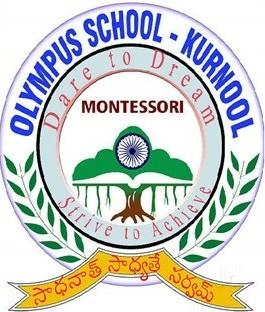# Montessori Olympus School Quiz

10 Questions | Total Attempts: 26SettingsCreate your own QuizTopic : Light Prepared by N. Prashanth (Physics Teacher-7396328685)

• 1.
According to fermat principle, the route which light travel from A to B
• A.

Route 2

• B.

Route 1

• C.

Route 4

• D.

Route 3

• 2.
From this figure i =
• A.

500

• B.

900

• C.

400

• D.

1800

• 3.
From this figure the principal axis is
• A.

AR

• B.

AC

• C.

AP

• D.

PC

• 4.
1. By extension of reflected rays we can get ___________ image
• A.

Virtual Image

• B.

Real Image

• C.

Inverted Image

• D.

No image

• 5.
Among these which is not a rule of sign convention?
• A.

Measure all distances from centre of curvature

• B.

The distance in light travelling direction is positive

• C.

Distance in opposite direction is negative

• D.

​​​​​​Measure all distances from (Pole)

• 6.
If radius of curvature of a spherical mirror (R) = 14cm them the focal length =____________
• A.

28 cm

• B.

35 cm

• C.

7 cm

• D.

21 cm

• 7.
Focal laugh (f) =-15cm object distence (u) = -25cm height of the object (ho) = +4cm then the image is
• A.

Enlarged

• B.

Inverted

• C.

Both Enlarged & Inverted

• D.

None of these

• 8.
In mirrors the virtual image is formed when the object is at _____________
• A.

Focus

• B.

​​​​​​between pole and focus

• C.

At centre of curvature

• D.

Beyond the centre of curvature

• 9.
The image of an object in pinhole camera is ________________
• A.

Erect

• B.

Inverted

• C.

Diminished

• D.

Plain

• 10.
In plane mirrors, right - left inversion of image is called
• A.

Inverse

• B.

Reverse

• C.

Lateral Inverse

• D.

Same side

Related TopicsBack to top
×

Wait!
Here's an interesting quiz for you.